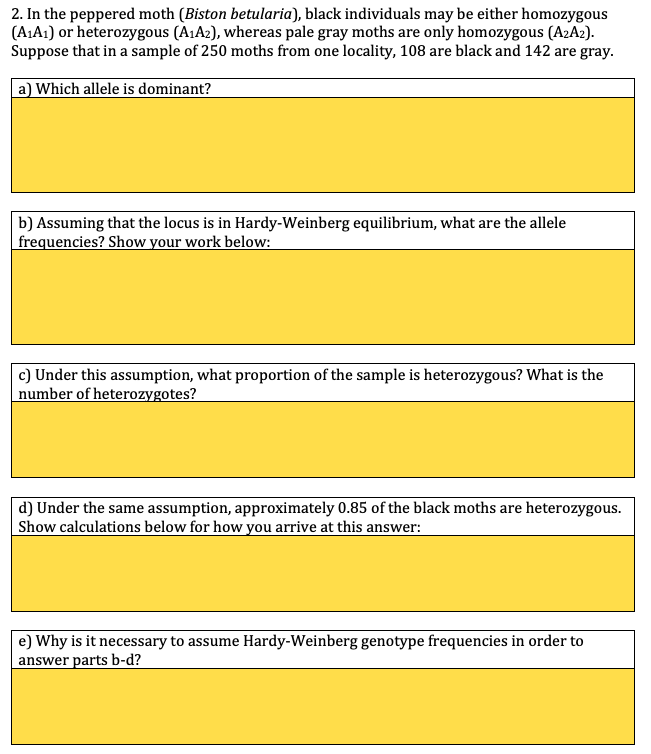# Solved: 2. In The Peppered Moth (Biston Betularia), Black Individuals May Be Either Homozygous (A1A1) Or Heterozygous (A1A2), Whereas Pale Gray Moths Are Only Homozygous (A2A2) Suppose That In A Sampl

By |will rate

2. In the peppered moth (Biston betularia), black individuals may be either homozygous (A1A1) or heterozygous (A1A2), whereas pale gray moths are only homozygous (A2A2) Suppose that in a sample of 250 moths from one locality, 108 are black and 142 are gray a) Which allele is dominant? b) Assuming that the locus is in Hardy-Weinberg equilibrium, what are the allele frequencies? Show your work below: c) Under this assumption, what proportion of the sample is heterozygous? What is the number of heterozygotes? d) Under the same assumption, approximately 0.85 of the black moths are heterozygous Show calculations below for how you arrive at this answer: e) Why is it necessary to assume Hardy-Weinberg genotype frequencies in order to answer parts b-d?